# 1. A mass is connected to two springs with spring constants ki and k2, as shown....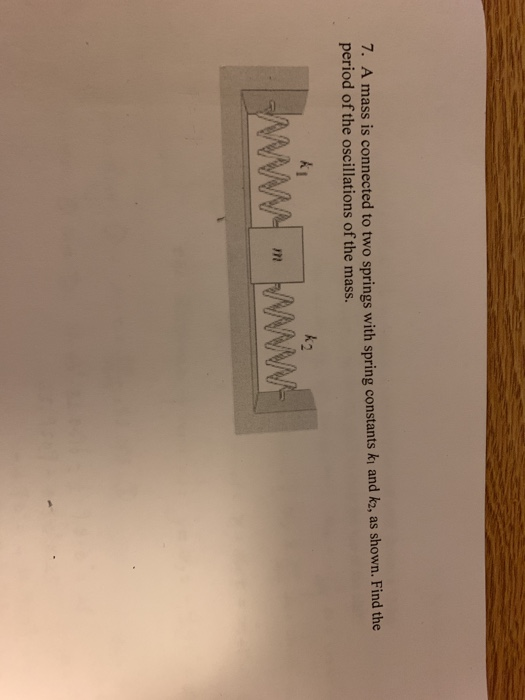1. A mass is connected to two springs with spring constants ki and k2, as shown. Find the period of the oscillations of the mass. Wwm WWW-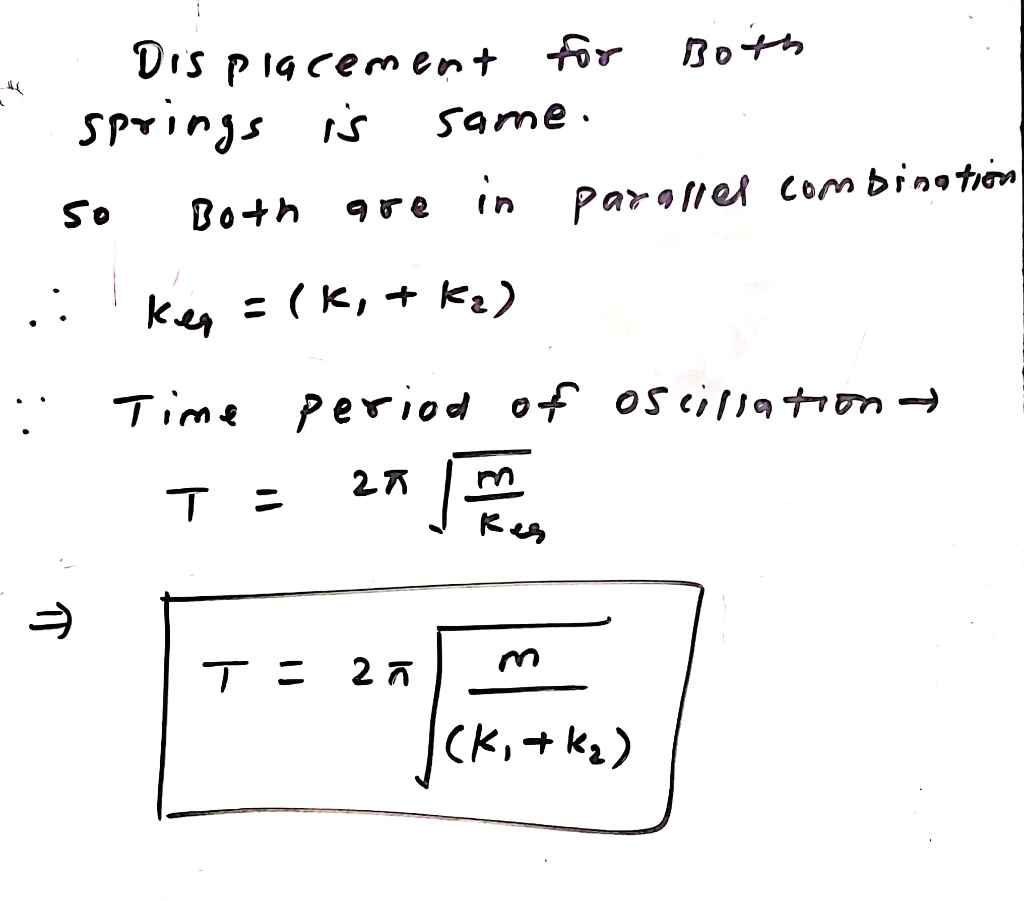#### Earn Coin

Coins can be redeemed for fabulous gifts.

Similar Homework Help Questions
• ### Question: A block with mass of m = 3.78 kg is attached to springs with spring...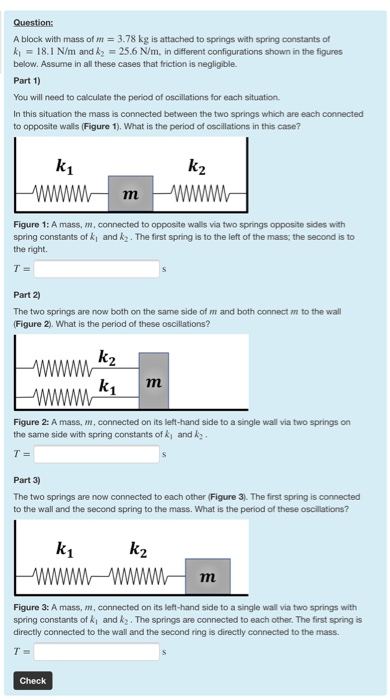Question: A block with mass of m = 3.78 kg is attached to springs with spring constants of ki = 18.1 N/m and k = 25.6 N/m, in different configurations shown in the figures below. Assume in all these cases that friction is negligible. Part 1) You will need to calculate the period of oscillations for each situation In this situation the mass is connected between the two springs which are each connected to opposite walls (Figure 1). What is...

• ### Two springs, with force constants k1=150N/m and k2=235N/m, are connected in series Two springs, with force...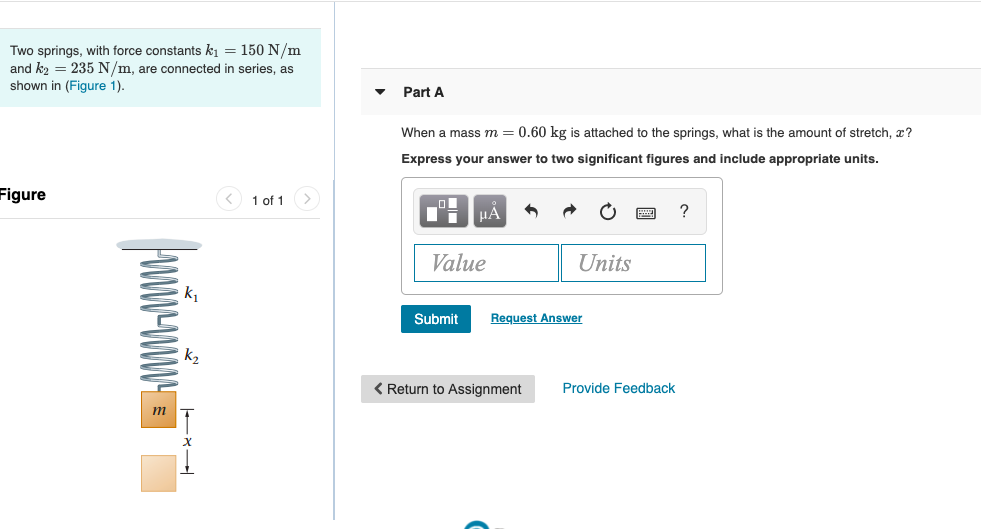Two springs, with force constants k1=150N/m and k2=235N/m, are connected in series Two springs, with force constants ki = 150 N/m and k2 = 235 N/m, are connected in series, as shown in (Figure 1). Part A When a mass m = 0.60 kg is attached to the springs, what is the amount of stretch, ? Express your answer to two significant figures and include appropriate units. Figure < 1 of 1 > TT HÀ • • • Ea ?...

• ### A hinged rigid bar is connected by two springs of stiffnesses kı and k2 and is subjected to a for...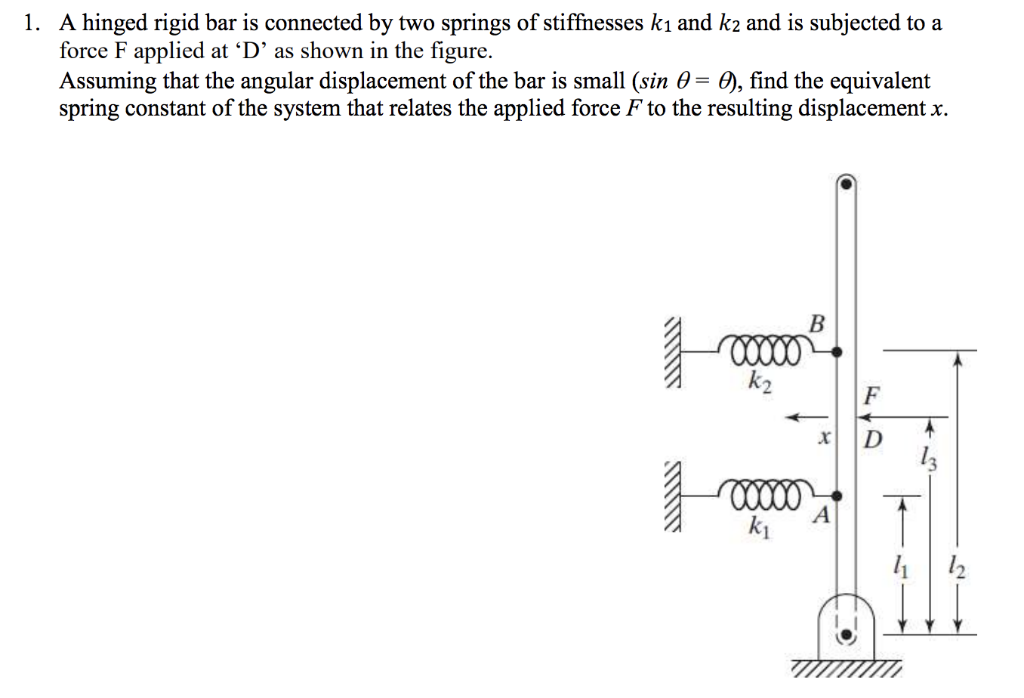A hinged rigid bar is connected by two springs of stiffnesses kı and k2 and is subjected to a force F applied at 'D' as shown in the figure. Assuming that the angular displacement of the bar is small (sin θ-6), find the equivalent spring constant of the system that relates the applied force F to the resulting displacement x. 1. ki A hinged rigid bar is connected by two springs of stiffnesses kı and k2 and is subjected to...

• ### Newton's Third Law (two springs) Two springs with spring constants k1 = 24.6 N/m and k2...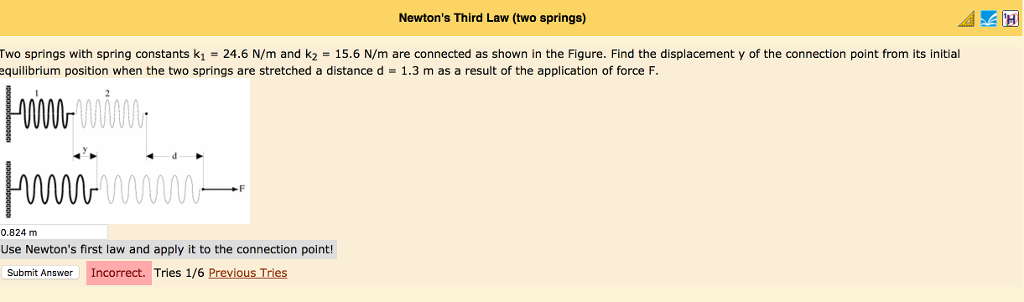Newton's Third Law (two springs) Two springs with spring constants k1 = 24.6 N/m and k2 = 15.6 N/m are connected as shown in the Figure. Find the displacement y of the connection point from its initial equilibrium position when the two springs are stretched a distance d = 1.3 m as a result of the application of force F 0 0.824 m Use Newton's first law and apply it to the connection point! Submit Answer Incorrect. Tries 1/6 Previous...

• ### Two Springs of constants ky and are connected in series to a block A that vibrates...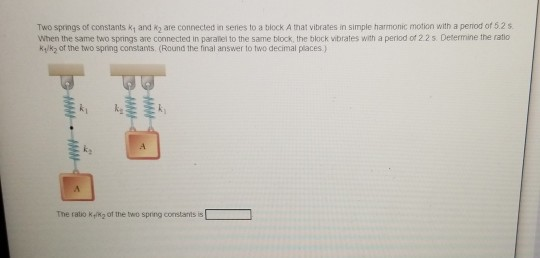Two Springs of constants ky and are connected in series to a block A that vibrates in simple harmonic motion with a period of 52 When the same two Springs are connected in parallel to the same block, the block vibrates with a period of 22 s. Determine the ratio Kyk of the two spring constants (Round the final answer to two decimal places) WWW . w The ratio of the two spring constant is

• ### 4. Two masses mi and m2 are connected to three springs of negligible mass having spring constants...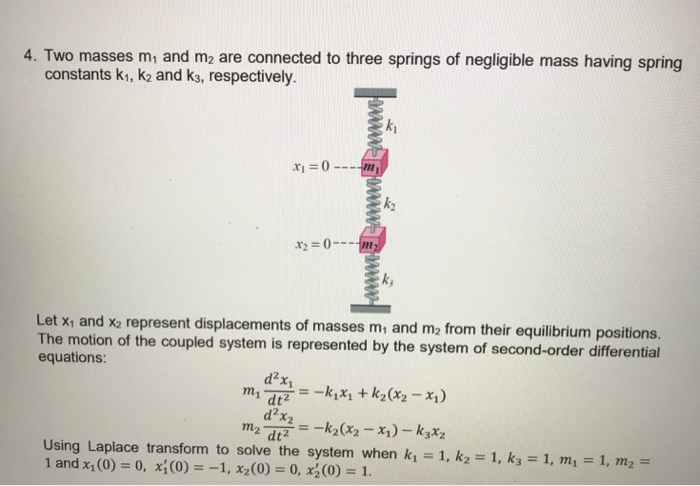4. Two masses mi and m2 are connected to three springs of negligible mass having spring constants k1, k2 and k3, respectively. x2=0 Il k, Let xi and x2 represent The motion of the equations: displacements of masses mi and m2 from their equilibrium positions . coupled system is represented by the system of second-order differential d2x dt2 d2x2 Using Laplace transform to solve the system when k1 1 and x1(0) = 0, xi (0)--1 , x2(0) = 0, x(0)-1....

• ### Two springs, with force constants k1=170N/m and k2=240N/m, are connected in series. When a mass m=0.55kg...

Two springs, with force constants k1=170N/m and k2=240N/m, are connected in series. When a mass m=0.55kg is attached to the springs, what is the amount of stretch, x?

• ### 3. Consider the spring - mass system shown below, consisting of two masses mi and m2 sus- pended ...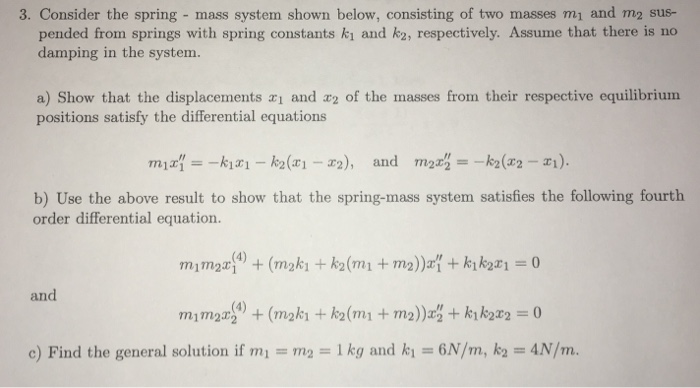3. Consider the spring - mass system shown below, consisting of two masses mi and m2 sus- pended from springs with spring constants ki and k2, respectively. Assume that there is no damping in the system. a) Show that the displacements ai and r2 of the masses from their respective equilibrium positions satisfy the differential equations b) Use the above result to show that the spring-mass system satisfies the following fourth order differential equation and c) Find the general solution...

• ### number 6 solve Two springs with spring constants k_1 and k_2, are connected with each other...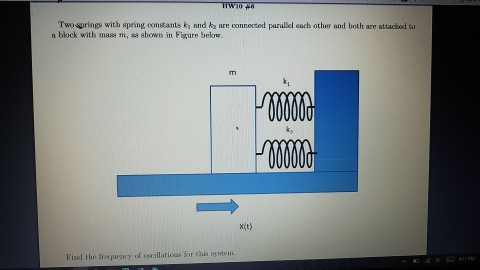number 6 solve Two springs with spring constants k_1 and k_2, are connected with each other and attached to a block with mass m, as shown in Figure below.

• ### Three springs with spring constants ki =40N/m, ky =10N/m, and K3 =40N/m are connected in SERIES....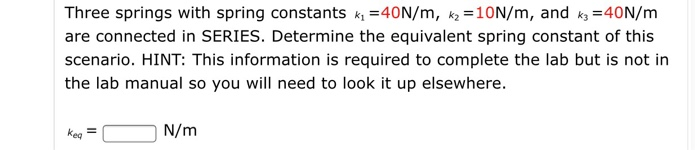Three springs with spring constants ki =40N/m, ky =10N/m, and K3 =40N/m are connected in SERIES. Determine the equivalent spring constant of this scenario. HINT: This information is required to complete the lab but is not in the lab manual so you will need to look it up elsewhere. keq= N/m